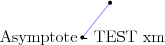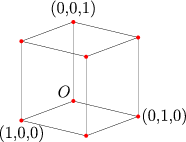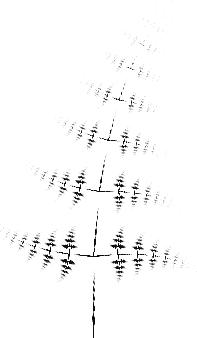Difference between revisions of "User talk:WikiSysop"

Test Asymptote

Test October 27th [asy] draw((0,0)--(3,7),red); dot((0,0)); dot((3,7)); label("Produced with Asymptote "+version.VERSION,point(S),2S); [/asy]Test Cite Extension

Example: Cite-Extension

Test MathJax

\begin{align} \dot{x} & = \sigma(y-x) \\ \dot{y} & = \rho x - y - xz \\ \dot{z} & = -\beta z + xy \end{align}

$\frac{1}{(\sqrt{\phi \sqrt{5}}-\phi) e^{\frac25 \pi}} = 1+\frac{e^{-2\pi}} {1+\frac{e^{-4\pi}} {1+\frac{e^{-6\pi}} {1+\frac{e^{-8\pi}} {1+\ldots} } } }$

Some Text $$\frac{1}{(\sqrt{\phi \sqrt{5}}-\phi) e^{\frac25 \pi}} = 1+\frac{e^{-2\pi}} {1+\frac{e^{-4\pi}} {1+\frac{e^{-6\pi}} {1+\frac{e^{-8\pi}} {1+\ldots} } } }$$

Some Text $\frac{1}{(\sqrt{\phi \sqrt{5}}-\phi) e^{\frac25 \pi}} = 1+\frac{e^{-2\pi}} {1+\frac{e^{-4\pi}} {1+\frac{e^{-6\pi}} {1+\frac{e^{-8\pi}} {1+\ldots} } } }$

Pages A-Z

Alphabetically ordered index of all pages

Recent Changes

List of previous changes on EOM

.

How to Cite This Entry:
WikiSysop. Encyclopedia of Mathematics. URL: http://www.encyclopediaofmath.org/index.php?title=WikiSysop&oldid=34088

Test January 12th 2015